### Dividing Indices

#### Here the rules used are:

am ÷ an = am − n   and    a1 = a

#### Here the rules demonstrated are:

a− n = 1/an   and    a0 = 1

#### Example 1.   work out   a7/a4

In this example the base is a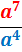==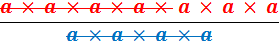= a × a × a = a7 − 4    =    a3

#### Example 2.   work out   a4/a4

In this example the base is a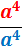=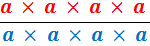== a4 − 4    =    a0    =    1

Remember any number divided by itself is equal to 1; this is because any number multiplied by 1 is equal to that same number.

#### Example 3.   work out 10a6/2a3

In this example the base is a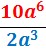=== 5a × a × a = 5a6 − 3    =    5a3

#### Example 4.   work out a3/a7

In this example the base is a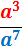=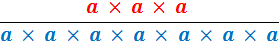==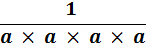= a3 − 7    =    a−4 =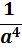#### Example 5.   work out 12a2/6a5

In this example the base is a==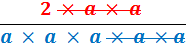=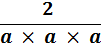=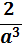#### Example 6.   work out 12a/3a6

In this example the base is a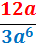=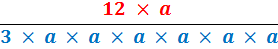=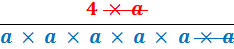==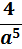to: